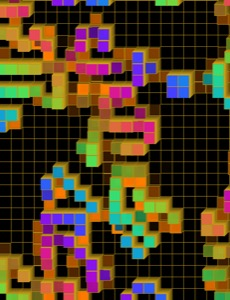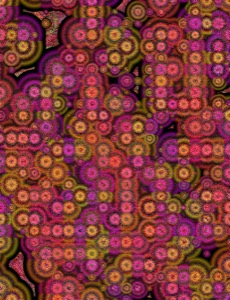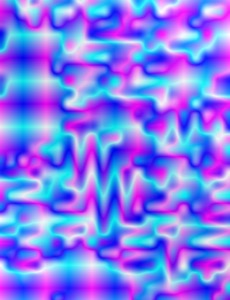# Conway's life variation

### Notes on a shader based on life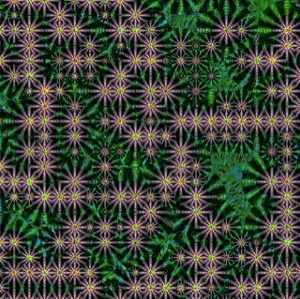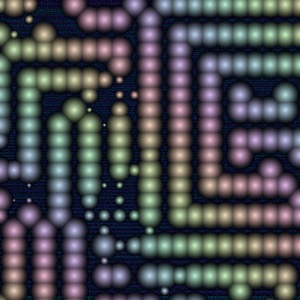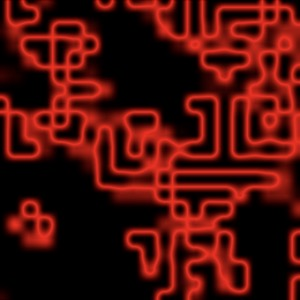Conway invented his famous game of life in 1970. There are many many web pages and articles devoted to this incredible game, e.g., ConwayLife.com and Wikipedia page. There is a vast and thriving community around investigating constructions and phenomena associated with this game.
I decided to hang some shader art work on a variation of the game of life here. On this page I describe what I did. This is an elementary low tech description. A few example screenshots are shown.

## Art based on Conway's game of life

### Rendering. Notes on Shaders/colouring algorithm

[[a,b],[c,d]]
 0.25 0.75 1 0

a=A b=A
c=A d=A

### Overlapping tiles

In the above example, the values in the array of life array each control a separate tile. But this means there can't be any overlapping of the images, because different tiles are not passed any information about what is in neighbouring tiles. (I mean in a WebGl situation where they are not passed more info. The above picture is svg, for less code) To overlap tiles, we will have to pass more information. E.g., instead of passing just the value of A to tile at position (0,0), we could pass the values in A, A, A, A to the tile at the (0,0) position. The most basic case would be just to have the values used to control four different things, e.g., have four quarter circles, one of each of these radii, at each of the four corners.
In the following picure, the info from 9 cells in the JavaScript array is passed to 4 tiles. Each tile gets the data from 4 cells. In order to show that the cells are distinct, I move them slightly apart, but in the program I am describing here, the squares match up exactly. Also in the example here, four pieces of data are passed to each tile. The top left gets the data a=A, b=A, c=A, d=A; the top right gets a=A, b=A, c=A, d=A; The bottom left gets a=A, b=A, c=A, d=A; the bottom right gets a=A, b=A, c=A, d=A. In some of the examples in the shader I'm describing, I pass information from the nearest corresponding 16 cells in a 4x4 region.
A A A
A A A
A A A
[[a,b,c],[d,e,f],[g,h,i]]
 a d g b e h c f i

Description: Each tile gets parameters a,b,c,d from the array A, as described above.
For circles, these determine the radius of circles centered at each of the four corners of the tile. The circles can be coloured so that the colours match, but to emphasise that the same code is used with different variables in the different tiles, I have used the same colouring per tile. i.e, red top left, blue top right, green bottom left, yellow bottom right.
For Voronoi the colour of a point in a tile is determined by the vertex it is closest to - except the distance is weighted, so instead of comparing the distance from the point to each of the four corners of the tile, we multiply the distance by the value assigned to the corner by a, b, c, or d, depending on the corner.
For rgba a,b,c,d determine respectively the red, green, blue and opacity values of the colour of the tile.
For mix the shade is given by a number determined by a mix of the colours assigned to each corner of the tile. The closer to a corner, the closer the shade is to the value assigned to that corner. There are many different ways to mix values in a continuous way, I choose a very simple option, taken from the book of shaders.
For folds I use the same mixing formula to give a value for a point on the tile, then I apply shading that instead of going from black to white as the parameter goes from 0 to 1, alternates from black to white more frequently.
The button option uses the parameters to draw a picture of parts of four buttons, with the different values of the parameter determining the values of the buttons's height.